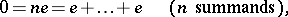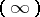# Characteristic of a field

(diff) ← Older revision | Latest revision (diff) | Newer revision → (diff)
A positive prime number or the number 0 that is uniquely determined for a given field in the following way. If for some,whereis the unit element of the field, then the smallest suchis a prime number; it is called the characteristic of. If there are no such numbers, then one says that the characteristic ofis zero or thatis a field of characteristic zero. Sometimes such a field is said to be without characteristic or of characteristic infinity. Every field of characteristic zero contains a subfield isomorphic to the field of all rational numbers, and a field of finite characteristiccontains a subfield isomorphic to the field of residue classes modulo.